Related Articles

# How to use Color Palettes in Python-Bokeh?

• Last Updated : 28 Jul, 2020

Bokeh is a Python interactive data visualization. It renders its plots using HTML and JavaScript. It targets modern web browsers for presentation providing elegant, concise construction of novel graphics with high-performance interactivity. Bokeh provides us with multiple color palettes in the `bokeh.palettes` module. Let us see how to use these color palettes in Bokeh.

A palette is a simple plain Python list of (hex) RGB color strings. For example, the `blues8` palette has the colors : `('#084594', '#2171b5', '#4292c6', '#6baed6', '#9ecae1', '#c6dbef', '#deebf7', '#f7fbff')`.

There are 5 types of built-in color palettes in Bokeh :

## Matplotlib Palettes

Bokeh provides us with Matplotlib color palettes. There are 5 types of Matplotlib color palettes :

• Magma
• Inferno
• Plasma
• Viridis
• Cividis

Each type of color palette has 10 different versions of the palette with varying number of colors, which are 3, 4, 5, 6, 7, 8, 9, 10, 11 and 256.

Example : We will be demonstrating the Matplotlib palettes by plotting multiple vertical bars using the vbar() function.

 `# importing the modules ``from` `bokeh.plotting ``import` `figure, output_file, show ``from` `bokeh.palettes ``import` `Magma, Inferno, Plasma, Viridis, Cividis`` ` `# file to save the model ``output_file(``"gfg.html"``) ``        ` `# instantiating the figure object ``graph ``=` `figure(title ``=` `"Bokeh Palettes"``) `` ` `# demonstrating the Magma palette``graph.vbar(x ``=` `[``1``, ``2``, ``3``, ``4``, ``5``, ``6``, ``7``, ``8``, ``9``, ``10``, ``11``], ``           ``top ``=` `[``9``] ``*` `11``,``           ``bottom ``=` `[``8``] ``*` `11``,``           ``width ``=` `1``,``           ``color ``=` `Magma[``11``])`` ` `# demonstrating the Inferno palette``graph.vbar(x ``=` `[``1``, ``2``, ``3``, ``4``, ``5``, ``6``, ``7``, ``8``, ``9``, ``10``, ``11``], ``           ``top ``=` `[``7``] ``*` `11``,``           ``bottom ``=` `[``6``] ``*` `11``,``           ``width ``=` `1``,``           ``color ``=` `Inferno[``11``])`` ` `# demonstrating the Plasma palette``graph.vbar(x ``=` `[``1``, ``2``, ``3``, ``4``, ``5``, ``6``, ``7``, ``8``, ``9``, ``10``, ``11``], ``           ``top ``=` `[``5``] ``*` `11``,``           ``bottom ``=` `[``4``] ``*` `11``,``           ``width ``=` `1``,``           ``color ``=` `Plasma[``11``])`` ` `# demonstrating the Viridis palette``graph.vbar(x ``=` `[``1``, ``2``, ``3``, ``4``, ``5``, ``6``, ``7``, ``8``, ``9``, ``10``, ``11``], ``           ``top ``=` `[``3``] ``*` `11``,``           ``bottom ``=` `[``2``] ``*` `11``,``           ``width ``=` `1``,``           ``color ``=` `Viridis[``11``])`` ` `# demonstrating the Cividis palette``graph.vbar(x ``=` `[``1``, ``2``, ``3``, ``4``, ``5``, ``6``, ``7``, ``8``, ``9``, ``10``, ``11``], ``           ``top ``=` `[``1``] ``*` `11``,``           ``width ``=` `1``,``           ``color ``=` `Cividis[``11``])``    ` `# displaying the model ``show(graph)`

Output :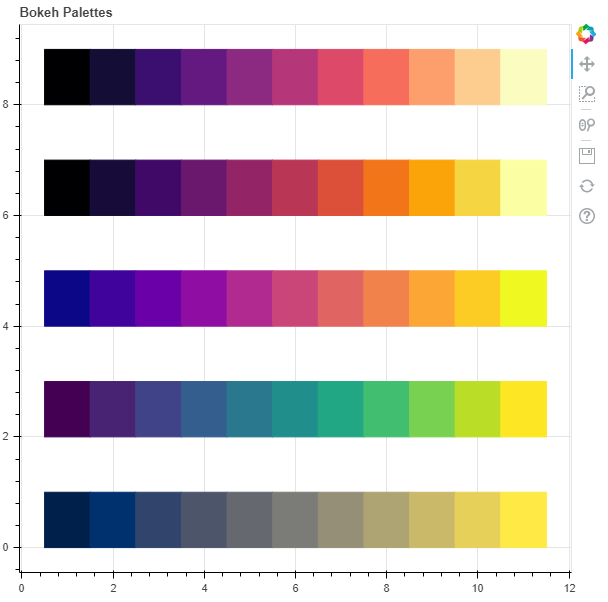## D3 Palettes

Bokeh provides us with D3 categorical color palettes. There are 4 types of D3 color palettes available :

• Category10
• Category20
• Category20b
• Category20c

Example : We will be demonstrating the D3 palettes by plotting multiple vertical bars using the vbar() function.

 `# importing the modules ``from` `bokeh.plotting ``import` `figure, output_file, show ``from` `bokeh.palettes ``import` `Category10, Category20, Category20b, Category20c`` ` `# file to save the model ``output_file(``"gfg.html"``) ``        ` `# instantiating the figure object ``graph ``=` `figure(title ``=` `"Bokeh Palettes"``) `` ` `# demonstrating the Category10 palette``graph.vbar(x ``=` `[``1``, ``2``, ``3``, ``4``, ``5``, ``6``, ``7``, ``8``, ``9``, ``10``], ``           ``top ``=` `[``9``] ``*` `10``,``           ``bottom ``=` `[``8``] ``*` `10``,``           ``width ``=` `1``,``           ``color ``=` `Category10[``10``])`` ` `# demonstrating the Category20 palette``graph.vbar(x ``=` `[``1``, ``2``, ``3``, ``4``, ``5``, ``6``, ``7``, ``8``, ``9``, ``10``], ``           ``top ``=` `[``7``] ``*` `10``,``           ``bottom ``=` `[``6``] ``*` `10``,``           ``width ``=` `1``,``           ``color ``=` `Category20[``10``])`` ` `# demonstrating the Category20b palette``graph.vbar(x ``=` `[``1``, ``2``, ``3``, ``4``, ``5``, ``6``, ``7``, ``8``, ``9``, ``10``], ``           ``top ``=` `[``5``] ``*` `10``,``           ``bottom ``=` `[``4``] ``*` `10``,``           ``width ``=` `1``,``           ``color ``=` `Category20b[``10``])`` ` `# demonstrating the Category20c palette``graph.vbar(x ``=` `[``1``, ``2``, ``3``, ``4``, ``5``, ``6``, ``7``, ``8``, ``9``, ``10``], ``           ``top ``=` `[``3``] ``*` `10``,``           ``bottom ``=` `[``2``] ``*` `10``,``           ``width ``=` `1``,``           ``color ``=` `Category20c[``10``])`` ` `# displaying the model ``show(graph)`

Output :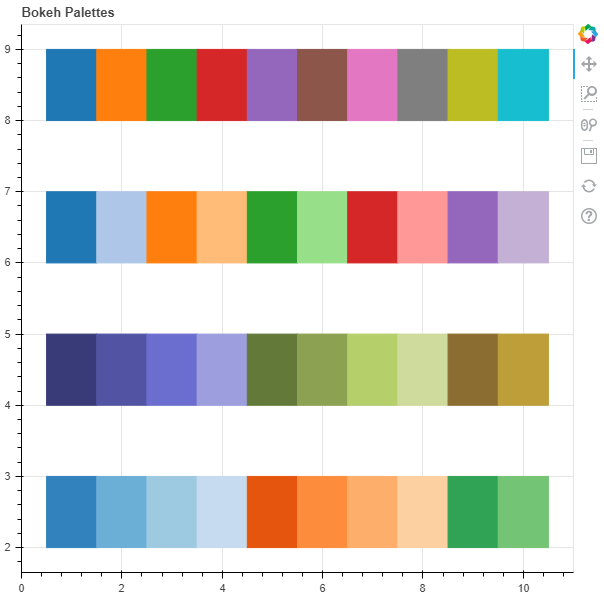## Brewer Palettes

Bokeh provides us with ColorBrewer palettes. There are 35 types of ColorBrewer palettes available :

• Accent
• Blues
• BrBG
• BuGn
• BuPu
• Dark2
• GnBu
• Greens
• Greys
• OrRd
• Oranges
• PRGn
• Paired
• Pastel1
• Pastel2
• PiYG
• PuBu
• PuBuGn
• PuOr
• PuRd
• Purples
• RdBu
• RdGy
• RdPu
• RdYlBu
• RdYlGn
• Reds
• Set1
• Set2
• Set3
• Spectral
• YlGn
• YlGnBu
• YlOrBr
• YlOrRd

Example : We will be demonstrating the ColorBrewer palettes by plotting multiple vertical bars using the vbar() function.

 `# importing the modules ``from` `bokeh.plotting ``import` `figure, output_file, show ``from` `bokeh.palettes ``import` `BrBG, PiYG, RdGy, RdYlGn, YlGnBu`` ` `# file to save the model ``output_file(``"gfg.html"``) ``        ` `# instantiating the figure object ``graph ``=` `figure(title ``=` `"Bokeh Palettes"``) `` ` `# demonstrating the BrBG palette``graph.vbar(x ``=` `[``1``, ``2``, ``3``, ``4``, ``5``, ``6``, ``7``, ``8``, ``9``], ``           ``top ``=` `[``9``] ``*` `9``,``           ``bottom ``=` `[``8``] ``*` `9``,``           ``width ``=` `1``,``           ``color ``=` `BrBG[``9``])`` ` `# demonstrating the PiYG palette``graph.vbar(x ``=` `[``1``, ``2``, ``3``, ``4``, ``5``, ``6``, ``7``, ``8``, ``9``], ``           ``top ``=` `[``7``] ``*` `9``,``           ``bottom ``=` `[``6``] ``*` `9``,``           ``width ``=` `1``,``           ``color ``=` `PiYG[``9``])`` ` `# demonstrating the RdGy palette``graph.vbar(x ``=` `[``1``, ``2``, ``3``, ``4``, ``5``, ``6``, ``7``, ``8``, ``9``], ``           ``top ``=` `[``5``] ``*` `9``,``           ``bottom ``=` `[``4``] ``*` `9``,``           ``width ``=` `1``,``           ``color ``=` `RdGy[``9``])`` ` `# demonstrating the RdYlGn palette``graph.vbar(x ``=` `[``1``, ``2``, ``3``, ``4``, ``5``, ``6``, ``7``, ``8``, ``9``], ``           ``top ``=` `[``3``] ``*` `9``,``           ``bottom ``=` `[``2``] ``*` `9``,``           ``width ``=` `1``,``           ``color ``=` `RdYlGn[``9``])`` ` `# demonstrating the YlGnBu palette``graph.vbar(x ``=` `[``1``, ``2``, ``3``, ``4``, ``5``, ``6``, ``7``, ``8``, ``9``], ``           ``top ``=` `[``1``] ``*` `9``,``           ``width ``=` `1``,``           ``color ``=` `YlGnBu[``9``])`` ` `# displaying the model ``show(graph) `

Output :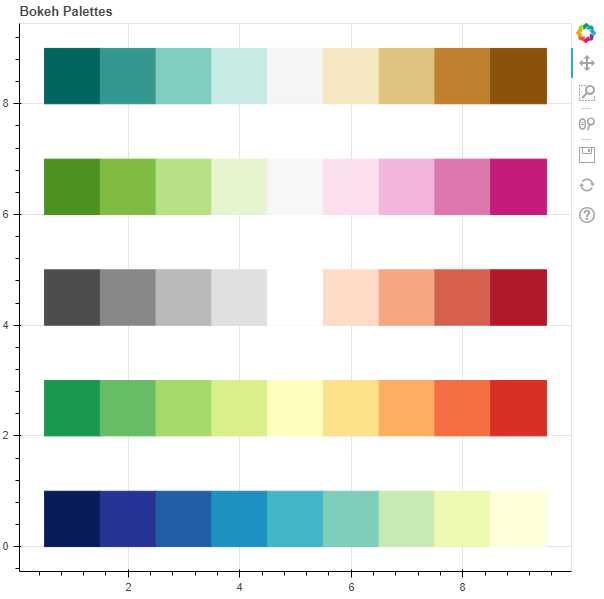## Usability Palettes

Bokeh provides us with a palette that is useful for people with color deficiency or color blindness.

Example : We will be demonstrating the usability palette by plotting multiple vertical bars using the vbar() function.

 `# importing the modules ``from` `bokeh.plotting ``import` `figure, output_file, show ``from` `bokeh.palettes ``import` `Colorblind`` ` `# file to save the model ``output_file(``"gfg.html"``) ``        ` `# instantiating the figure object ``graph ``=` `figure(title ``=` `"Bokeh Palettes"``) `` ` `# demonstrating the Colorblind palette``graph.vbar(x ``=` `[``1``, ``2``, ``3``, ``4``, ``5``, ``6``, ``7``, ``8``], ``           ``top ``=` `[``1``] ``*` `8``,``           ``width ``=` `1``,``           ``color ``=` `Colorblind[``8``])`` ` `# displaying the model ``show(graph)`

Output :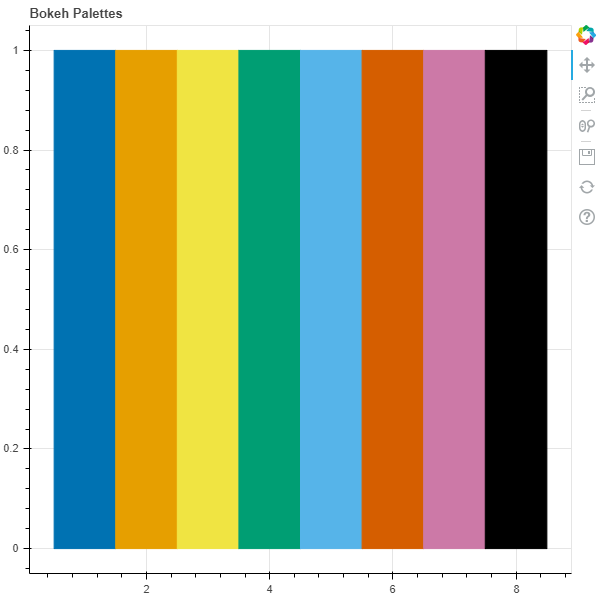## Large Palettes

The color palettes discussed above might be small for some applications. Bokeh provides us with large palettes that have 256 colors each. There are 7 large palettes :

• Greys256
• Inferno256
• Magma256
• Plasma256
• Viridis256
• Cividis256
• Turbo256

Example : We will be demonstrating the large palettes by plotting multiple vertical bars using the vbar() function.

 `# importing the modules ``from` `bokeh.plotting ``import` `figure, output_file, show ``from` `bokeh.palettes ``import` `Greys256, Inferno256, Magma256, Plasma256``from` `bokeh.palettes ``import` `Viridis256, Cividis256, Turbo256`` ` `# file to save the model ``output_file(``"gfg.html"``) ``        ` `# instantiating the figure object ``graph ``=` `figure(title ``=` `"Bokeh Palettes"``) `` ` `# demonstrating the Greys256 palette``graph.vbar(x ``=` `[i ``for` `i ``in` `range``(``256``)], ``           ``top ``=` `[``20``] ``*` `256``,``           ``bottom ``=` `[``18``] ``*` `256``,``           ``width ``=` `1``,``           ``color ``=` `Greys256)`` ` `# demonstrating the Inferno256 palette``graph.vbar(x ``=` `[i ``for` `i ``in` `range``(``256``)], ``           ``top ``=` `[``17``] ``*` `256``,``           ``bottom ``=` `[``15``] ``*` `256``,``           ``width ``=` `1``,``           ``color ``=` `Inferno256)`` ` `# demonstrating the Magma256 palette``graph.vbar(x ``=` `[i ``for` `i ``in` `range``(``256``)], ``           ``top ``=` `[``14``] ``*` `256``,``           ``bottom ``=` `[``12``] ``*` `256``,``           ``width ``=` `1``,``           ``color ``=` `Magma256)`` ` `# demonstrating the Plasma256 palette``graph.vbar(x ``=` `[i ``for` `i ``in` `range``(``256``)], ``           ``top ``=` `[``11``] ``*` `256``,``           ``bottom ``=` `[``9``] ``*` `256``,``           ``width ``=` `1``,``           ``color ``=` `Plasma256)`` ` `# demonstrating the Viridis256 palette``graph.vbar(x ``=` `[i ``for` `i ``in` `range``(``256``)], ``           ``top ``=` `[``8``] ``*` `256``,``           ``bottom ``=` `[``6``] ``*` `256``,``           ``width ``=` `1``,``           ``color ``=` `Viridis256)`` ` `# demonstrating the Cividis256 palette``graph.vbar(x ``=` `[i ``for` `i ``in` `range``(``256``)], ``           ``top ``=` `[``5``] ``*` `256``,``           ``bottom ``=` `[``3``] ``*` `256``,``           ``width ``=` `1``,``           ``color ``=` `Cividis256)`` ` `# demonstrating the Turbo256 palette``graph.vbar(x ``=` `[i ``for` `i ``in` `range``(``256``)], ``           ``top ``=` `[``2``] ``*` `256``,``           ``bottom ``=` `[``0``] ``*` `256``,``           ``width ``=` `1``,``           ``color ``=` `Turbo256)`` ` `# displaying the model ``show(graph) `

Output :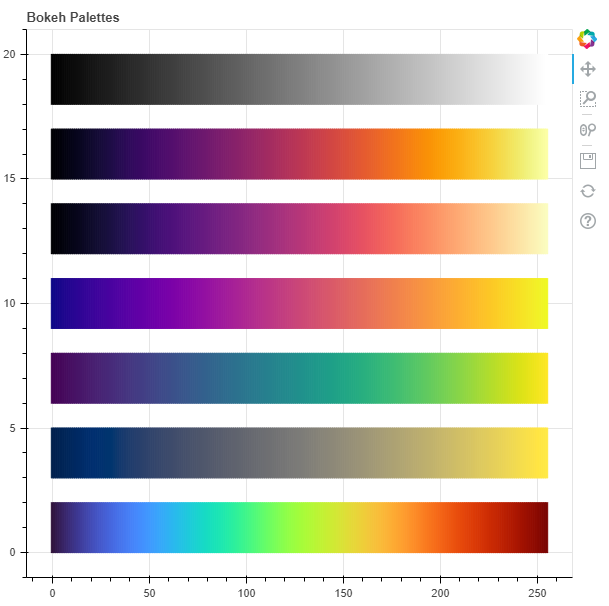Attention geek! Strengthen your foundations with the Python Programming Foundation Course and learn the basics.

To begin with, your interview preparations Enhance your Data Structures concepts with the Python DS Course. And to begin with your Machine Learning Journey, join the Machine Learning – Basic Level Course

My Personal Notes arrow_drop_up